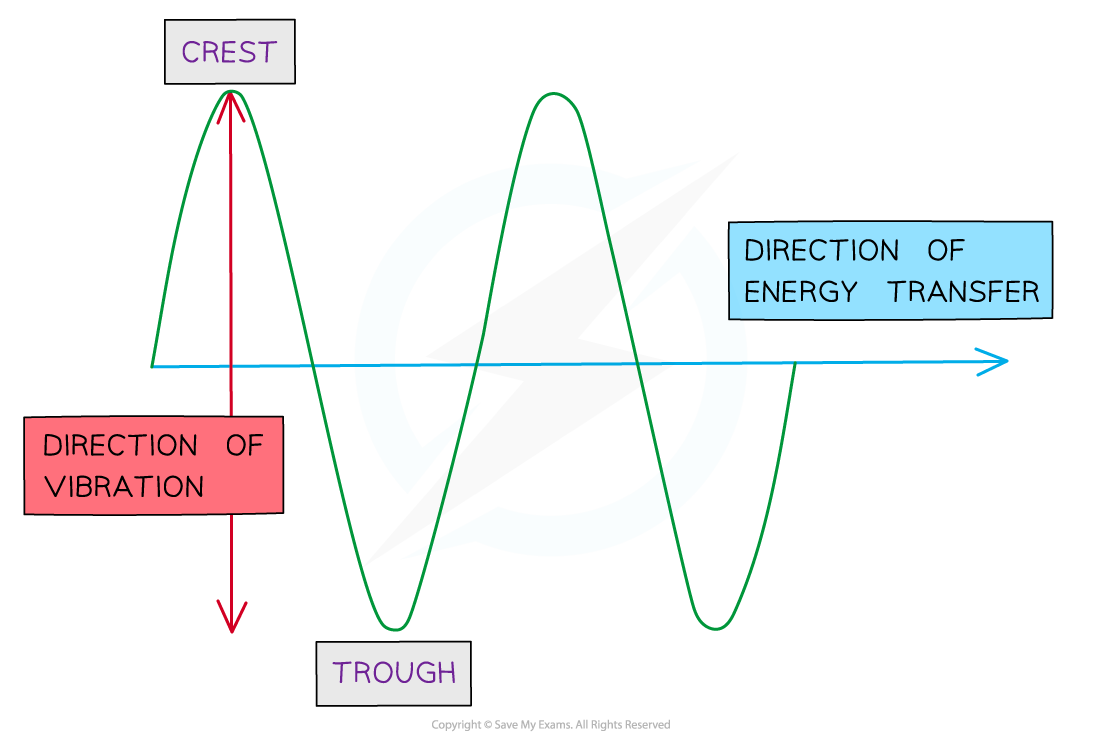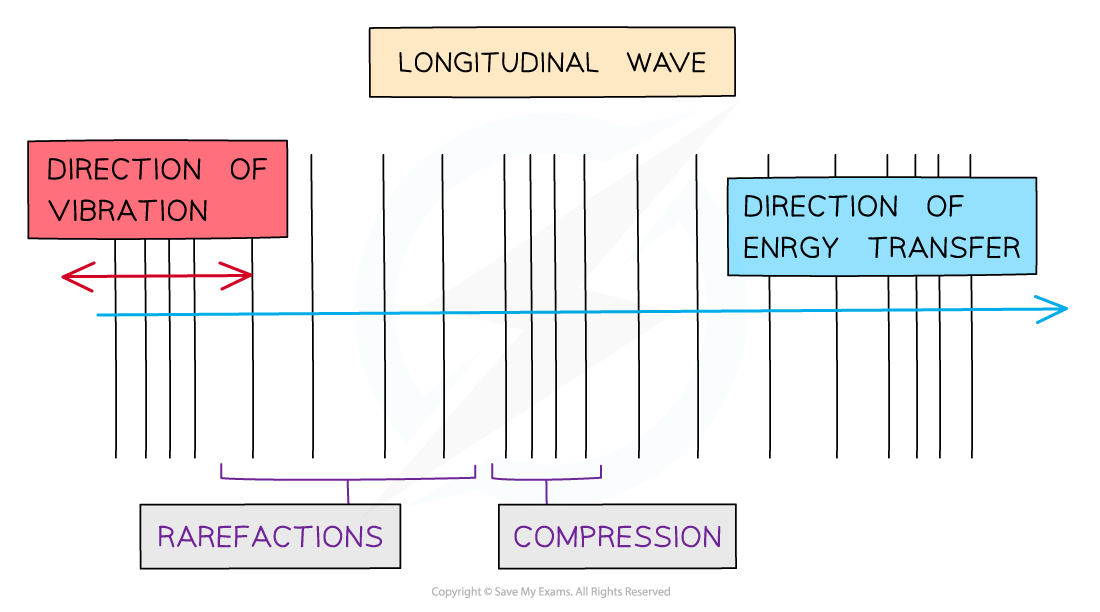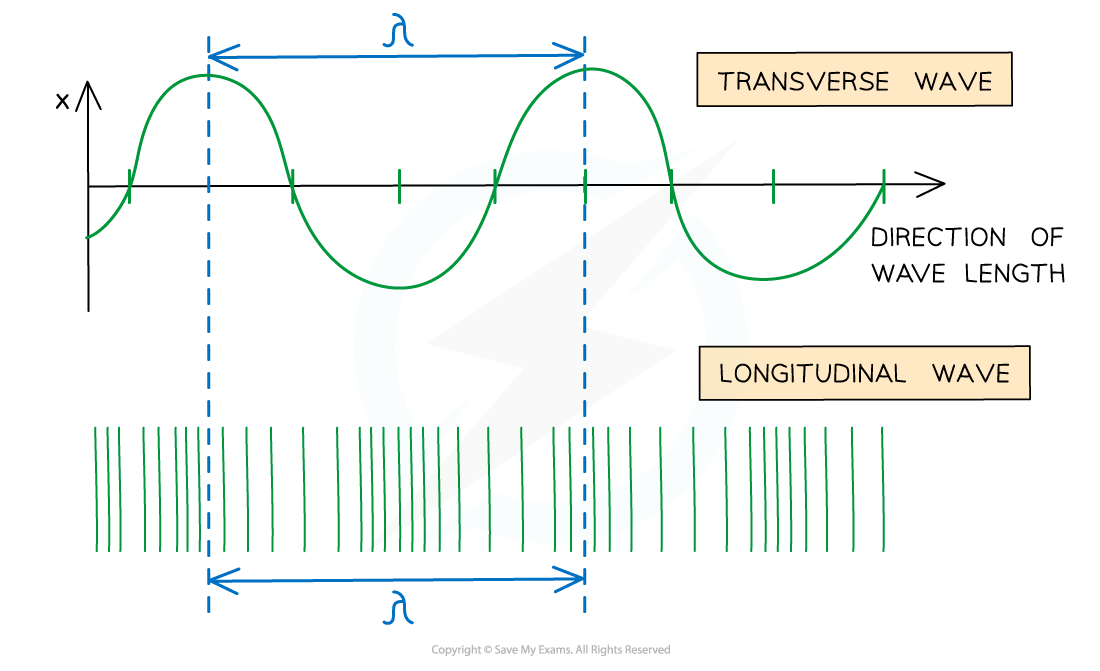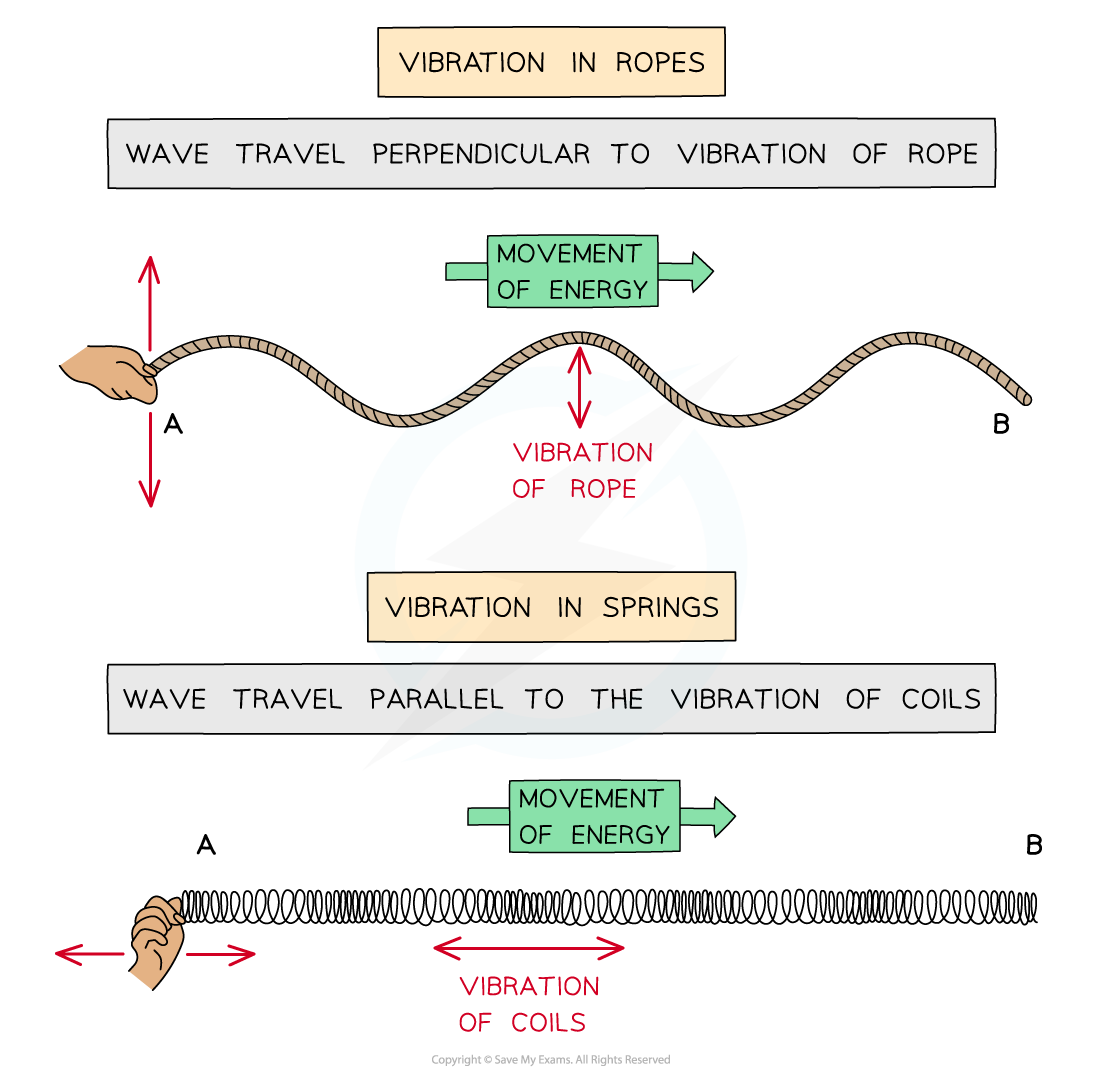# AQA A Level Physics复习笔记3.1.2 Longitudinal & Transverse Waves

### Defining Transverse & Longitudinal Waves

• In mechanical waves, particles oscillate about fixed points
• There are two types of wave: transverse and longitudinal
• The type of wave can be determined by the direction of the oscillations in relation to the direction the wave is travelling

#### Transverse Waves

• A transverse wave is defined as:

A wave in which the particles oscillate perpendicular to the direction of the wave travel (and energy transfer)

• Transverse waves show areas of crests (peaks) and troughsDiagram of a transverse wave

• Examples of transverse waves are:
• Electromagnetic waves e.g. radio, visible light, UV
• Vibrations on a guitar string

• Transverse waves can be shown on a rope
• Transverse waves can be polarised

#### Longitudinal Waves

• A longitudinal wave is defined as:

A wave in which the particles oscillate parallel to the direction of the wave travel (and energy transfer)

• Longitudinal waves show areas of compressions and rarefactions
• Compressions are regions of increased pressure
• Rarefactions are regions of decreased pressureDiagram of a longitudinal wave

• Examples of longitudinal waves are:
• Sound waves
• Ultrasound waves

• Longitudinal waves can be shown on a slinky spring
• Longitudinal waves cannot be polarised
• Energy is transmitted through the wave by:
• The particles in the medium vibrating as they are given energy
• The compressions cause the nearby particles to also vibrate with more energy
• This produces a compression further along in the mediumA wavelength on a longitudinal wave is the distance between two compressions or two rarefactions

#### Exam Tip

The definition of transverse and longitudinal waves are often asked as exam questions, make sure to remember these definitions by heart!

### Examples of Transverse & Longitudinal Waves

• Energy is transferred through moving oscillations or vibrations. These can be seen in vibrations of ropes or springsWaves can be shown through vibrations in ropes or springs

• The oscillations / vibrations can be perpendicular or parallel to the direction of wave travel:
• Waves which oscillate in a perpendicular direction are transverse waves
• Waves which oscillate in a parallel direction are longitudinal waves

• Examples of transverse waves are:
• Electromagnetic waves e.g. radio, visible light, UV
• Vibrations on a guitar string
• Waves on a string
• Seismic (S) waves

• Examples of longitudinal waves are:
• Sound waves
• Ultrasound waves
• Waves through a slinky coil
• Seismic (P) waves